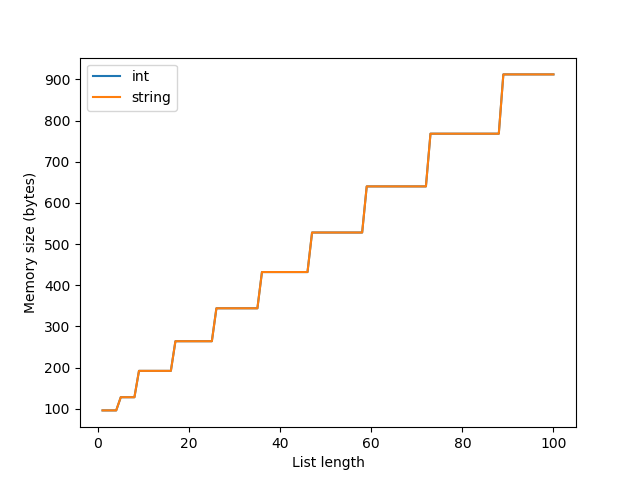31 July 2019

How much memory does a python list require? This is easy to answer. Python has a function called getsizeof that will tell you directly:

``````>>> from sys import getsizeof
>>> getsizeof([1,2,3])
88
``````

Okay, great, thanks Python! But why does this list take up 88 bytes? Also, if you run:

``````from sys import getsizeof
test_str = []
test_int = []
for i in range(100):
test_int.append(i)
test_str.append(str(i))
print(i, getsizeof(test_int), getsizeof(test_str))
``````

and plot the results, you’ll get:Why does a list of integers have the same reported size as a list of strings, when ‘1’ is larger than 1?

``````>>> getsizeof('1')
50
>>> getsizeof(1)
28
``````

Why does the size increase in steps at a time, instead of strictly linear?

In the cpython listobject.c list_resize code, you can see that the memory for a list is allocated using the integer variable new_allocated :

``````    num_allocated_bytes = new_allocated * sizeof(PyObject *);
items = (PyObject **)PyMem_Realloc(self->ob_item, num_allocated_bytes);
``````

new_allocated is calculated using this formula:

``````    new_allocated = (size_t)newsize + (newsize >> 3) + (newsize < 9 ? 3 : 6);
``````

newsize here is the requested resize of the list. When using append, this is going to be len(list)+1. new_allocated is always going to be larger than new_size. If you append to a list, and new_size is less than or equal to the already allocated size, the function returns early and memory is not reallocated. This is a time-saving feature; if you’re appending to a list, there’s a good chance you’re going to append to it again soon, so it doesn’t make sense to do the computationally expensive step of reallocating memory every time. This is why there’s a discrete rather than continuous trend between list size and allocated memory.

Let’s go over this step by step:

• [].append(0) (1 + 1 » 3 + (1 < 9 ? 3 : 6) => (1 + 0 + 3) => 4
• .append(1) 2<=4, do nothing => 4
• [0, 1].append(2) 3<=4, do nothing => 4
• [0, 1, 2].append(3) 4<=4, do nothing => 4
• [0, 1, 2, 3].append(4) (5 + 5 » 3 + (5 < 9 ? 3 : 6) => (5 + 0 + 3) => 8

and so on.

We can also see that list_resize is allocating the size of a PyObject pointer, rather than the size of the object that you are appending to the list. Each item in a list exists elsewhere in memory, and the list just contains pointers to those items. If you want the true size of a list in python, you need to do recursively descend through each item in the list.

Finally, if you look at the cpython source code for getsizeof, you will see:

``````    if (PyObject_IS_GC(o))
``````

getsizeof adds on the memory cost of garbage collection. So, finally, the breakdown of that 88 bytes for the size of [1,2,3] on 64 bit systems is:

• 40 bytes for the size of the list PyObject
• 4 allocated size * 6 byte size of a PyObject pointer = 24
• 24 bytes for garbage collection

On 32 bit systems, this is:

• 20 bytes for the size of the list PyObject
• 4 allocated size * 4 byte size of a PyObject pointer = 16
• 12 bytes for garbage collection

Wow, garbage collection is expensive! Examining why is a separate post.